隐马尔科夫模型(HMM)

马尔科夫链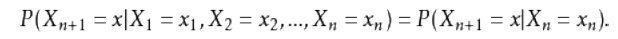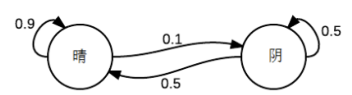(10)[0.90.50.10.5]=(0.90.1)$(1 \quad 0) *\begin{equation} { \left[ \begin{array}{ccc} 0.9 & 0.1 \\ 0.5 & 0.5 \end{array} \right ] } \end{equation}=(0.9 \quad 0.1)$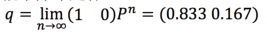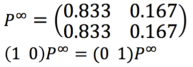1. 状态：晴天、阴天
2. 初始向量：定义系统在时间为0的时候的状态的概率
3. 状态转移矩阵：每种天气转换的概率。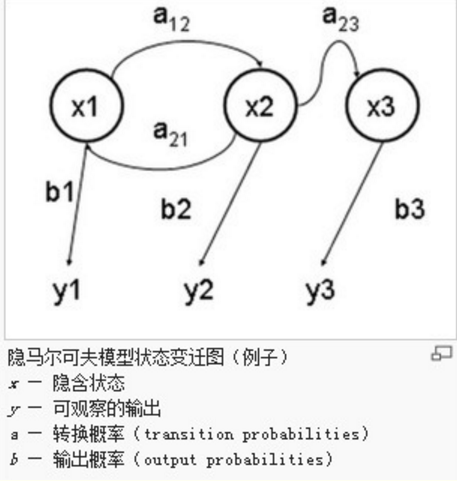隐马尔可夫链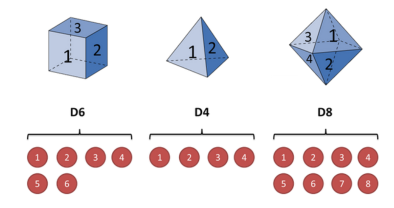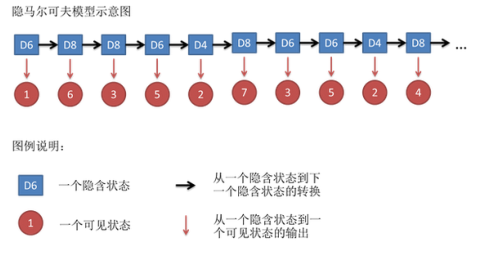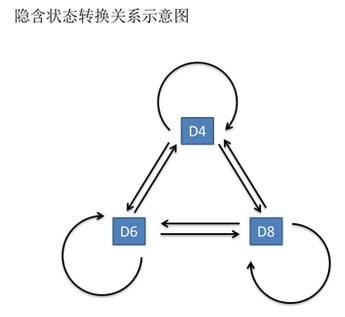1. 知道骰子有几种（隐含状态数量），每种骰子是什么（转换概率），根据掷骰子掷出的结果（可见状态链），我想知道每次掷出来的都是哪种骰子（隐含状态链）。 在语音识别领域呢，叫做解码问题。
2. 还是知道骰子有几种（隐含状态数量），每种骰子是什么（转换概率），根据掷骰子掷出的结果（可见状态链），我想知道掷出这个结果的概率。 -反欺诈。
3. 知道骰子有几种（隐含状态数量），不知道每种骰子是什么（转换概率），观测到很多次掷骰子的结果（可见状态链），我想反推出每种骰子是什么（转换概率）。

1. Recognition：Given the observation sequence O=O1O2...OT$O=O_1O_2...O_T$ and a model λ=A,B,π$λ=（A,B,π）$，how do we choose a corresponding state sequence Q=q1q2...qT$Q=q_1q_2...q_T$ which is optimal in some sense, i.e.,best explains the observations
2. Evaluation：Given the observation sequence O=O1O2...OT$O=O_1O_2...O_T$ and a mode λ=A,B,π$λ=（A,B,π）$, how do we efficiently compute P(O|λ)$P(O|λ)$, i.e.,the probability of the observation sequence given the model
3. Training：Given the observation sequence O=O1O2...OT$O=O_1O_2...O_T$ , how do we adjust the model parameters λ=A,B,π$λ=（A,B,π）$ to maximize P(O|λ)$P(O|λ)$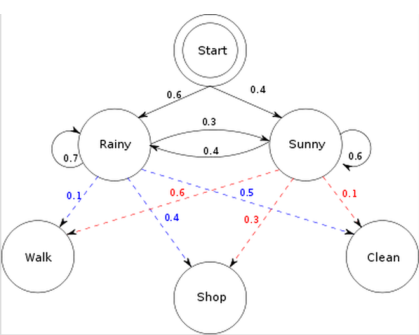1. 已知整个模型，我观测到连续三天做的事情是：散步，购物，收拾，我想猜，这三天的天气是怎么样的。
2. 已知整个模型，我观测到连续三天做的事情是：散步，购物，收拾。那么根据模型，计算产生这些行为的概率是多少。
3. 最复杂的，我只知道这三天做了这三件事儿，而其他什么信息都没有。我得建立一个模型，晴雨转换概率，第一天天气情况的概率分布，根据天气情况选择做某事的概率分布。

1. 问题一：Viterbi Algo，维特比算法。
2. 问题二：Forword Algorithm，向前算法，或者 Backward Algorithm，向后算法。
3. 问题三：Baum-Welch Algo，鲍姆-韦尔奇算法

• 找到概率最大的隐含序列，意味着计算 argmaxQP(Q|O,λ)$argmax_QP(Q|O,λ)$ ,相当于计算 argmaxQP(QO|λ)$argmax_QP(Q，O|λ)$
• Viterbi 算法（动态规划）定义 δj(t)$\delta_j(t)$，保存在时间t时，观测值和状态Sj$S_j$ 的最大可能性
• 归纳：
•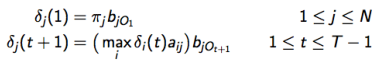• 使用回溯（backtracking）（保持最大参数对于每个t和j）来发现最优解

• 需要计算 P(O|λ)$P(O|λ)$ ，给出模型 λ 的条件下，可见序列为 O=O1O2...OT$O=O_1O_2...O_T$ 的可能性。
• 我们能够枚举所有可能的隐含状态序列 Q=q1q2...qT$Q=q_1q_2...q_T$
• 对于其中一个隐含状态序列 Q$Q$
•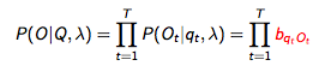• 对于这个隐含状态序列 Q$Q$ 的可能性是：
•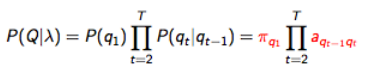• 因此联合可能性为：
•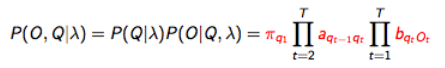• 考虑所有可能隐含状态序列
•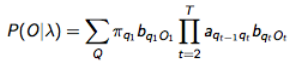• 存在问题：需要 2TNT$2TN^T$ 的计算量
• NT$N^T$的可能的隐含状态序列
• 每个序列需约 2T的计算量

• 定义前向变量 αj(t)$α_j(t)$ 作为 直到时间t ,和隐藏状态Sj$S_j$下，部分可见序列的可能性：
•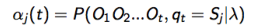• 可用归纳计算：
•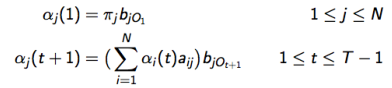• 通过 N2T$N^2T$ 复杂度的计算：
•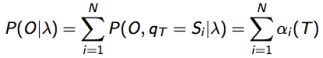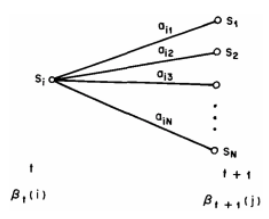• 定义后向变量 βi(t)$β_i(t)$ 作为时间t 之后，给定 t 时刻的隐藏状态Si$S_i$下，部分观测序列的可能性：
•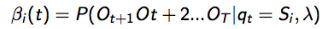• 归纳计算：
•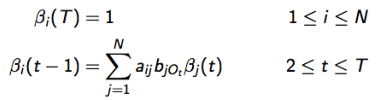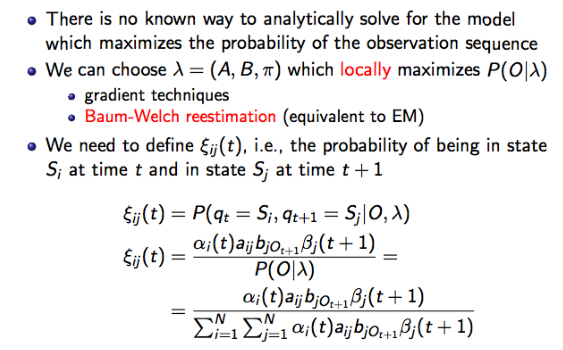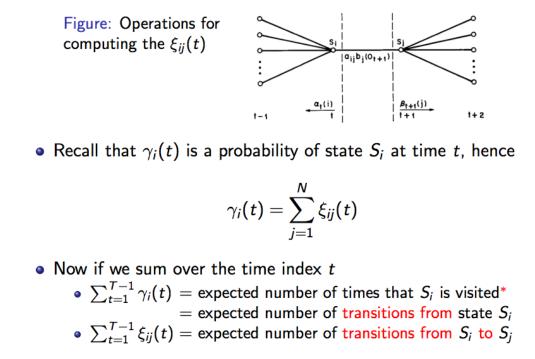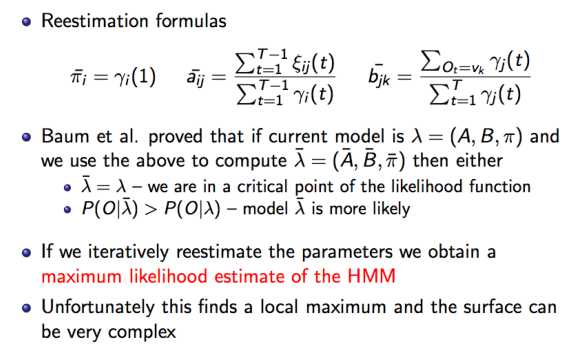隐马尔可夫链应用：词性标注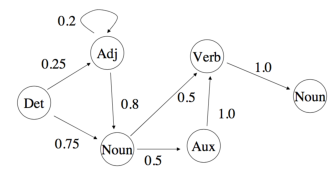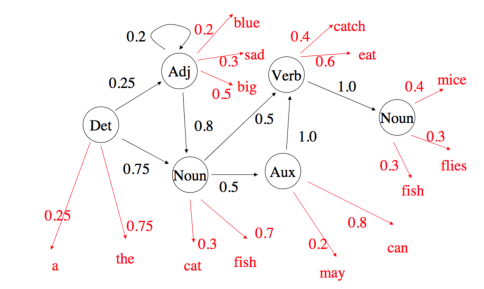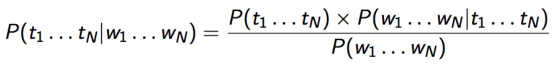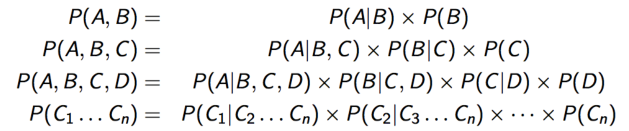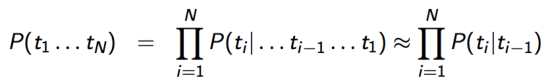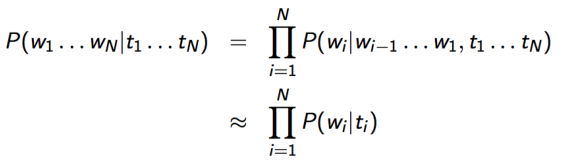• t1...tN$t_1...t_N$ 标签对应隐含状态 (zn)$(z_n)$
• w1...wN$w_1...w_N$ 词对应观察值 (xn)$(x_n)$
• 通过数 单词 和标签 我们能计算模型的参数
•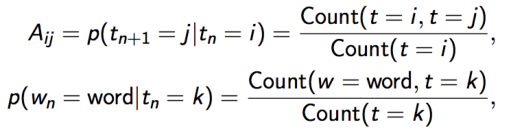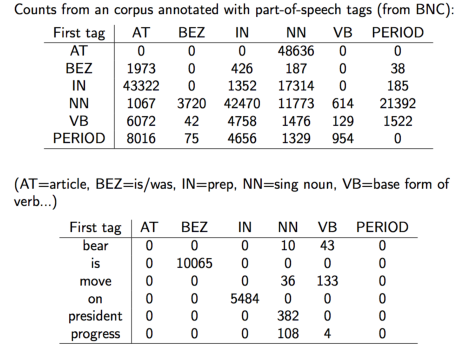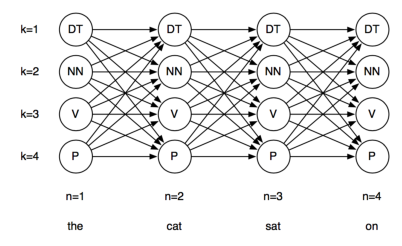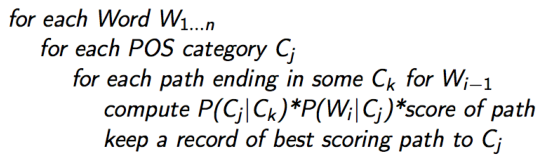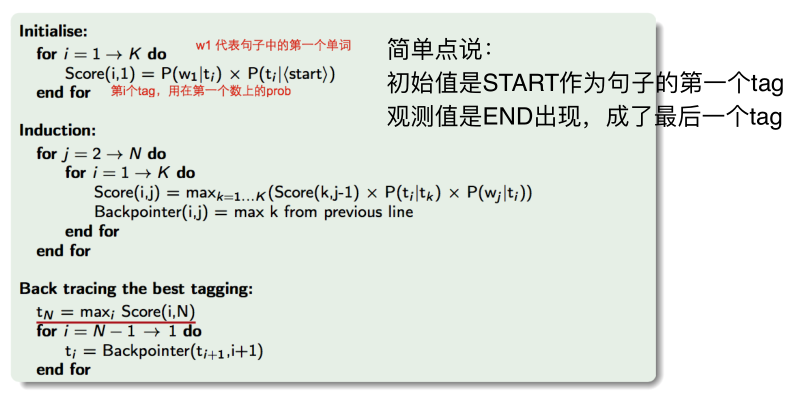使用HMM进行词性标注

Tag集： tags = t1...tN$t_1 ... t_N$

P(tags | words) 正比于 P(ti|ti1)P(wi|ti)$P(t_i | t_{i-1}) * P(w_i | t_i)$

import nltk
import sys
from nltk.corpus import brown

Brown 里面的句子都是自己标注好了的，长这个样子：（I,NOUN）,(LOVE,VERB),(YOU,NOUN)

brown_tags_words = [ ]
for sent in brown.tagged_sents():
# 先加开头
brown_tags_words.append( ("START", "START") )
# 为了省事儿，我们把tag都省略成前两个字母
brown_tags_words.extend([ (tag[:2], word) for (word, tag) in sent ])
# 加个结尾
brown_tags_words.append( ("END", "END") )

P(wi|ti)=count(wi,ti)count(ti)$P(w_i|t_i)=\frac{count(w_i,t_i)}{count(t_i)}$

# conditional frequency distribution
cfd_tagwords = nltk.ConditionalFreqDist(brown_tags_words)
# conditional probability distribution
cpd_tagwords = nltk.ConditionalProbDist(cfd_tagwords, nltk.MLEProbDist)

print("The probability of an adjective (JJ) being 'new' is", cpd_tagwords["JJ"].prob("new"))
print("The probability of a verb (VB) being 'duck' is", cpd_tagwords["VB"].prob("duck"))

The probability of an adjective (JJ) being ‘new’ is 0.01472344917632025
The probability of a verb (VB) being ‘duck’ is 6.042713350943527e-05

P(ti|ti1)=count(ti1,ti)count(ti1)$P(t_i|t_{i-1})=\frac{count(t_{i-1},t_i)}{count(t_{i-1})}$

brown_tags = [tag for (tag, word) in brown_tags_words ]
# count(t{i-1} ti)
# bigram的意思是 前后两个一组，联在一起
cfd_tags= nltk.ConditionalFreqDist(nltk.bigrams(brown_tags))
# P(ti | t{i-1})
cpd_tags = nltk.ConditionalProbDist(cfd_tags, nltk.MLEProbDist)

print("If we have just seen 'DT', the probability of 'NN' is", cpd_tags["DT"].prob("NN"))
print( "If we have just seen 'VB', the probability of 'JJ' is", cpd_tags["VB"].prob("DT"))
print( "If we have just seen 'VB', the probability of 'NN' is", cpd_tags["VB"].prob("NN"))

If we have just seen ‘DT’, the probability of ‘NN’ is 0.5057722522030194
If we have just seen ‘VB’, the probability of ‘JJ’ is 0.016885067592065053
If we have just seen ‘VB’, the probability of ‘NN’ is 0.10970977711020183

P(START) * P(PP|START) * P(I | PP) * P(VB | PP) * P(want | VB) * P(TO | VB) * P(to | TO) * P(VB | TO) * P(race | VB) * P(END | VB)

prob_tagsequence = cpd_tags["START"].prob("PP") * cpd_tagwords["PP"].prob("I") * \
cpd_tags["PP"].prob("VB") * cpd_tagwords["VB"].prob("want") * \
cpd_tags["VB"].prob("TO") * cpd_tagwords["TO"].prob("to") * \
cpd_tags["TO"].prob("VB") * cpd_tagwords["VB"].prob("race") * \
cpd_tags["VB"].prob("END")

print( "The probability of the tag sequence 'START PP VB TO VB END' for 'I want to race' is:", prob_tagsequence)

The probability of the tag sequence ‘START PP VB TO VB END’ for ‘I want to race’ is: 1.0817766461150474e-14

Viterbi 的实现

distinct_tags = set(brown_tags)

sentence = ["I", "want", "to", "race" ]
sentlen = len(sentence)

viterbi = [ ]

backpointer = [ ]
first_viterbi = { }
first_backpointer = { }
for tag in distinct_tags:
# don't record anything for the START tag
if tag == "START": continue
first_viterbi[ tag ] = cpd_tags["START"].prob(tag) * cpd_tagwords[tag].prob( sentence )
first_backpointer[ tag ] = "START"

print(first_viterbi)
print(first_backpointer)

{‘(-‘: 0.0, ‘UH’: 0.0, ‘EX’: 0.0, ‘–’: 0.0, ‘AP’: 0.0, ‘NI’: 3.3324520848931064e-07, “””: 0.0, ‘MD’: 0.0, ‘’: 0.0, ‘IN’: 0.0, ‘)’: 0.0, ‘CC’: 0.0, ‘WQ’: 0.0, ‘DT’: 0.0, ‘RB’: 0.0, ‘DO’: 0.0, ‘NP’: 1.7319067623793952e-06, ‘RP’: 0.0, ‘-‘: 0.0, ‘CS’: 0.0, ‘CD’: 0.0, ‘BE’: 0.0, ‘‘: 0.0, ‘FW’: 0.0, ‘END’: 0.0, ‘RN’: 0.0, ‘AT’: 0.0, ‘WD’: 0.0, ‘PN’: 0.0, ‘,-‘: 0.0, ‘,’: 0.0, ‘NR’: 0.0, “’”: 0.0, ‘:’: 0.0, ‘HV’: 0.0, ‘:-‘: 0.0, ‘TO’: 0.0, ‘)-‘: 0.0, ‘WR’: 0.0, ‘NN’: 1.0580313619573935e-06, ‘.’: 0.0, ‘OD’: 0.0, ‘WP’: 0.0, ‘(‘: 0.0, ‘PP’: 0.014930900689060006, ‘QL’: 0.0, ‘AB’: 0.0, ‘JJ’: 0.0, ‘.-‘: 0.0, ‘VB’: 0.0}
{‘(-‘: ‘START’, ‘UH’: ‘START’, ‘EX’: ‘START’, ‘–’: ‘START’, ‘AP’: ‘START’, ‘NI’: ‘START’, “””: ‘START’, ‘MD’: ‘START’, ‘“’: ‘START’, ‘IN’: ‘START’, ‘)’: ‘START’, ‘CC’: ‘START’, ‘WQ’: ‘START’, ‘DT’: ‘START’, ‘RB’: ‘START’, ‘DO’: ‘START’, ‘NP’: ‘START’, ‘RP’: ‘START’, ‘-‘: ‘START’, ‘CS’: ‘START’, ‘CD’: ‘START’, ‘BE’: ‘START’, ‘‘: ‘START’, ‘FW’: ‘START’, ‘END’: ‘START’, ‘RN’: ‘START’, ‘AT’: ‘START’, ‘WD’: ‘START’, ‘PN’: ‘START’, ‘,-‘: ‘START’, ‘,’: ‘START’, ‘NR’: ‘START’, “’”: ‘START’, ‘:’: ‘START’, ‘HV’: ‘START’, ‘:-‘: ‘START’, ‘TO’: ‘START’, ‘)-‘: ‘START’, ‘WR’: ‘START’, ‘NN’: ‘START’, ‘.’: ‘START’, ‘OD’: ‘START’, ‘WP’: ‘START’, ‘(‘: ‘START’, ‘PP’: ‘START’, ‘QL’: ‘START’, ‘AB’: ‘START’, ‘JJ’: ‘START’, ‘.-‘: ‘START’, ‘VB’: ‘START’}

viterbi.append(first_viterbi)
backpointer.append(first_backpointer)

currbest = max(first_viterbi.keys(), key = lambda tag: first_viterbi[ tag ])
print( "Word", "'" + sentence + "'", "current best two-tag sequence:", first_backpointer[ currbest], currbest)

Word ‘I’ current best two-tag sequence: START PP

for wordindex in range(1, len(sentence)):
this_viterbi = { }
this_backpointer = { }
prev_viterbi = viterbi[-1]

for tag in distinct_tags:
# START没有卵用的，我们要忽略
if tag == "START": continue

# 如果现在这个tag是X，现在的单词是w，
# 我们想找前一个tag Y，并且让最好的tag sequence以Y X结尾。
# 也就是说
# Y要能最大化：
# prev_viterbi[ Y ] * P(X | Y) * P( w | X)

best_previous = max(prev_viterbi.keys(),
key = lambda prevtag: \
prev_viterbi[ prevtag ] * cpd_tags[prevtag].prob(tag) * cpd_tagwords[tag].prob(sentence[wordindex]))

this_viterbi[ tag ] = prev_viterbi[ best_previous] * \
cpd_tags[ best_previous ].prob(tag) * cpd_tagwords[ tag].prob(sentence[wordindex])
this_backpointer[ tag ] = best_previous

# 每次找完Y 我们把目前最好的 存一下
currbest = max(this_viterbi.keys(), key = lambda tag: this_viterbi[ tag ])
print( "Word", "'" + sentence[ wordindex] + "'", "current best two-tag sequence:", this_backpointer[ currbest], currbest)

# 完结
# 全部存下来
viterbi.append(this_viterbi)
backpointer.append(this_backpointer)

Word ‘want’ current best two-tag sequence: PP VB
Word ‘to’ current best two-tag sequence: VB TO
Word ‘race’ current best two-tag sequence: IN NN

# 找所有以END结尾的tag sequence
prev_viterbi = viterbi[-1]
best_previous = max(prev_viterbi.keys(),
key = lambda prevtag: prev_viterbi[ prevtag ] * cpd_tags[prevtag].prob("END"))

prob_tagsequence = prev_viterbi[ best_previous ] * cpd_tags[ best_previous].prob("END")

# 我们这会儿是倒着存的。。。。因为。。好的在后面
best_tagsequence = [ "END", best_previous ]
# 同理 这里也有倒过来
backpointer.reverse()

current_best_tag = best_previous
for bp in backpointer:
best_tagsequence.append(bp[current_best_tag])
current_best_tag = bp[current_best_tag]

best_tagsequence.reverse()
print( "The sentence was:", end = " ")
for w in sentence: print( w, end = " ")
print("\n")
print( "The best tag sequence is:", end = " ")
for t in best_tagsequence: print (t, end = " ")
print("\n")
print( "The probability of the best tag sequence is:", prob_tagsequence)`

The sentence was: I want to race

The best tag sequence is: START PP VB IN NN END

The probability of the best tag sequence is: 5.71772824864617e-14